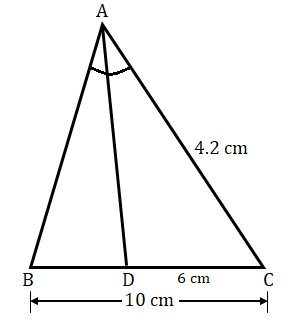"
">

# In a $Δ\ ABC$, $AD$ is the bisector of $∠\ A$, meeting side $BC$ at $D$. If $AC\ =\ 4.2\ cm$, $DC\ =\ 6\ cm$, and $BC\ =\ 10\ cm$, find $AB$."

Given:

In a $Δ\ ABC$, $AD$ is the bisector of $∠\ A$, meeting side $BC$ at $D$.

$AC\ =\ 4.2\ cm$, $DC\ =\ 6\ cm$, and $BC\ =\ 10\ cm$.

To do:

We have to find the measure of $AB$.

Solution:

From the figure,

$BC=BD+DC$

$BD=BC-DC=(10-6)\ cm=4\ cm$

$AD$ is the bisector of $∠\ A$, this implies,

$\angle BAD=\angle CAD$

We know that,

The internal bisector of an angle of a triangle divides the opposite side internally in the ratio of the corresponding sides containing the angle.

Therefore,

$\frac{AB}{AC} = \frac{BD}{DC}$

$\frac{AB}{4.2} = \frac{4}{6}$

$AB = \frac{4.2\times4}{6}$

$AB = \frac{8.4}{3}\ cm$

$AB=2.8\ cm$

The measure of $AB$ is $2.8\ cm$.

Updated on: 10-Oct-2022

27 Views